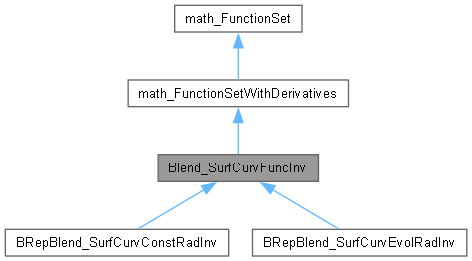Open CASCADE Technology  7.7.0.dev

# Blend_SurfCurvFuncInv Class Referenceabstract

Deferred class for a function used to compute a blending surface between a surface and a curve, using a guide line. This function is used to find a solution on a done restriction of the surface. More...

`#include <Blend_SurfCurvFuncInv.hxx>`

Inheritance diagram for Blend_SurfCurvFuncInv:[legend]

## Public Member Functions

Standard_Integer NbVariables () const
Returns 3. More...

virtual Standard_Integer NbEquations () const =0
returns the number of equations of the function. More...

virtual Standard_Boolean Value (const math_Vector &X, math_Vector &F)=0
computes the values <F> of the Functions for the variable <X>. Returns True if the computation was done successfully, False otherwise. More...

virtual Standard_Boolean Derivatives (const math_Vector &X, math_Matrix &D)=0
returns the values <D> of the derivatives for the variable <X>. Returns True if the computation was done successfully, False otherwise. More...

virtual Standard_Boolean Values (const math_Vector &X, math_Vector &F, math_Matrix &D)=0
returns the values <F> of the functions and the derivatives <D> for the variable <X>. Returns True if the computation was done successfully, False otherwise. More...

virtual void Set (const Handle< Adaptor2d_Curve2d > &Rst)=0
Set the Point on which a solution has to be found. More...

virtual void GetTolerance (math_Vector &Tolerance, const Standard_Real Tol) const =0
Returns in the vector Tolerance the parametric tolerance for each of the 3 variables; Tol is the tolerance used in 3d space. More...

virtual void GetBounds (math_Vector &InfBound, math_Vector &SupBound) const =0
Returns in the vector InfBound the lowest values allowed for each of the 3 variables. Returns in the vector SupBound the greatest values allowed for each of the 3 variables. More...

virtual Standard_Boolean IsSolution (const math_Vector &Sol, const Standard_Real Tol)=0
Returns Standard_True if Sol is a zero of the function. Tol is the tolerance used in 3d space. More...Public Member Functions inherited from math_FunctionSet
virtual Standard_Integer GetStateNumber ()
Returns the state of the function corresponding to the latestcall of any methods associated with the function. This function is called by each of the algorithms described later which define the function Integer Algorithm::StateNumber(). The algorithm has the responsibility to call this function when it has found a solution (i.e. a root or a minimum) and has to maintain the association between the solution found and this StateNumber. Byu default, this method returns 0 (which means for the algorithm: no state has been saved). It is the responsibility of the programmer to decide if he needs to save the current state of the function and to return an Integer that allows retrieval of the state. More...

virtual ~math_FunctionSet ()

## Detailed Description

Deferred class for a function used to compute a blending surface between a surface and a curve, using a guide line. This function is used to find a solution on a done restriction of the surface.

The vector <X> used in Value, Values and Derivatives methods has to be the vector of the parametric coordinates wguide, wcurv, wrst where wguide is the parameter on the guide line, wcurv is the parameter on the curve, wrst is the parameter on the restriction on the surface.

## ◆ Derivatives()

 virtual Standard_Boolean Blend_SurfCurvFuncInv::Derivatives ( const math_Vector & X, math_Matrix & D )
pure virtual

returns the values <D> of the derivatives for the variable <X>. Returns True if the computation was done successfully, False otherwise.

Implements math_FunctionSetWithDerivatives.

## ◆ GetBounds()

 virtual void Blend_SurfCurvFuncInv::GetBounds ( math_Vector & InfBound, math_Vector & SupBound ) const
pure virtual

Returns in the vector InfBound the lowest values allowed for each of the 3 variables. Returns in the vector SupBound the greatest values allowed for each of the 3 variables.

## ◆ GetTolerance()

 virtual void Blend_SurfCurvFuncInv::GetTolerance ( math_Vector & Tolerance, const Standard_Real Tol ) const
pure virtual

Returns in the vector Tolerance the parametric tolerance for each of the 3 variables; Tol is the tolerance used in 3d space.

## ◆ IsSolution()

 virtual Standard_Boolean Blend_SurfCurvFuncInv::IsSolution ( const math_Vector & Sol, const Standard_Real Tol )
pure virtual

Returns Standard_True if Sol is a zero of the function. Tol is the tolerance used in 3d space.

## ◆ NbEquations()

 virtual Standard_Integer Blend_SurfCurvFuncInv::NbEquations ( ) const
pure virtual

returns the number of equations of the function.

Implements math_FunctionSetWithDerivatives.

## ◆ NbVariables()

 Standard_Integer Blend_SurfCurvFuncInv::NbVariables ( ) const
virtual

Returns 3.

Implements math_FunctionSetWithDerivatives.

## ◆ Set()

 virtual void Blend_SurfCurvFuncInv::Set ( const Handle< Adaptor2d_Curve2d > & Rst )
pure virtual

Set the Point on which a solution has to be found.

## ◆ Value()

 virtual Standard_Boolean Blend_SurfCurvFuncInv::Value ( const math_Vector & X, math_Vector & F )
pure virtual

computes the values <F> of the Functions for the variable <X>. Returns True if the computation was done successfully, False otherwise.

Implements math_FunctionSetWithDerivatives.

## ◆ Values()

 virtual Standard_Boolean Blend_SurfCurvFuncInv::Values ( const math_Vector & X, math_Vector & F, math_Matrix & D )
pure virtual

returns the values <F> of the functions and the derivatives <D> for the variable <X>. Returns True if the computation was done successfully, False otherwise.

Implements math_FunctionSetWithDerivatives.